# Class 10 Science Worksheet for Electricity

Question 1
State Joule’s law of heating. list two special characteristics of a heating element wire.
An electronic iron consumed energy at the rate of 880 W when heating is at the maximum rate and 440 W when the heating is at the minimum rate. The applied voltage is 220 V. Calculate the current and resistance in each case

We know, $P = VI$
And $I= \frac {V}{R}$
So
$P= \frac {V^2}{R}$
$R = \frac {V^2}{P}$

Case Maximum Heating
At maximum heating, power is P = 840 W
Working voltage is 220 V
So, resistance at maximum heating is = (220)2/840 = 57.6 Ohm
Now I = P/V
So, current at maximum heating is = 840/220 = 3.8 A
Case Minimum Heating
At minimum heating, power is = 360 W
The resistance at minimum heating will be = (220)2/360 = 134.4 Ohm
The current at minimum heating will be = 360/220 = 1.6 A

Question 2
Copper and aluminium wires are usually employed for electricity transmission Why?

Copper and aluminium wires are usually employed for electricity transmission for the below reasons

(a)These are extremely good conductors
(b) They have a low value of resistivity.So while transmission over long distances, electricity is not dissipated in form of heat and energy is, hence, not wasted.
(c)these are ductile and can be drawn in the form of fine wire
(d) They are less expensive than the best conductors, silver and gold.
(e)They are extremely flexible and do not degrade under electrical transmission as do other metals.

Question 3
Obtain the expression for the heat developed in a resistor by the passage of electric current through it. 220 J of heat is produced each second in a 8 ohm resistor. Find the potential difference across the resistor.

(i) Let t be the time during which charge Q flows. Now when charge Q moves against the potential difference V , then the amount of work is given by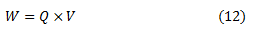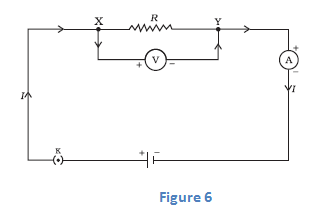Therefore the source must supply energy equal to VQ in time t. Hence power input to the circuit by the source isThe energy supplied to the circuit by the source in time t is P×t that is, VIt.
This is the amount of energy dissipated in the resistor as heat energy.
Thus for a steady current I flowing in the circuit for time t , the heat produced is given by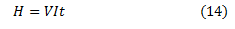Applying Ohm's law to above equation we get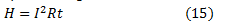This is known as Joule's Law of heating
ii) Now H=V2t/R
Here H=220J t=1 sec R=8 ohm
V2=1760
V=42 Volt

Question 4
(a) What is heating effect of current? List two electrical appliances which work on this effect.
(b) An electric bulb is connected to a 220 V generator. If the current drawn by the bulb is 0.50 A; find its power.
(c) An electric refrigerator rated 400 W operates eight hours a day. Calculate the energy per day in kWh.
(d) State the difference between kilowatt and kilowatt hour.
(e) Why is the series arrangement of appliances not used for domestic circuits?

(a) When electric current passes through a high resistance wire, the wire becomes and produces heat. This is called heating effect of current. electric Iron,Electric Heater are two appliances which work on this effect
(b) P=VI
=220X.50=110W
(c) Usage per day= 400 x 8 = 3200 Whr =3.2 KWh
(d) Kilowatt is measure of Power while Kilowatt hour is the measure of energy

Question 5
How much energy is given to each coulomb of charge passing through a 6 V battery?

Work done = Potential difference charge
Here P.D=6V and Q=1C
So work done=6J

Question 6
A copper wire has a diameter 0.5 mm and resistivity 1.6  10-8ohm m.
(i) What will be the length of this wire to make the resistance of 12 Ohm
(ii) How much will be the resistance of another copper wire of same length but half the diameter?

(a) Resistance of the wire is given by
$R=\rho \frac{l}{A}$
Here R=12 ohm,resistivity =1.6 X 10-8ohm m. And $A =\pi (d/2) ^2=\pi (.0005/2)^2$
So
$l= \frac{RA}{\rho}$
Substituting the above values
l=147.18 m
(b) Now when diameter is halved ,length remains same
$A =\pi (d/2) ^2=\pi (.00025/2)^2$ and l=147.18 m
$R=\rho \frac{l}{A}$
Substituting all the values we get
R=48 ohm

Question 7
A wire of uniform cross-section and length l has a resistance of 4 ohm. The wire is cut into four equal pieces. each piece is then stretched to length ‘l’. Thereafter, the four wires are joined in parallel. Calculate the net resistance

Let the resistivity of the material is ?, length of the wire initially l and the area of cross section is A. Then the resistance of the wire will be,
$R=\rho \frac{l}{A}=4$

Now cut the wire in equal four pieces and stretch it to the initial length l. When we stretch it the new length will be same of each piece but the area get reduced to one fourth ,So resistance of that piece would become
$R_1=\rho \frac{l}{A/4}=4\times 4=16$

Now, connect these four pieces in parallel. Then the equivalent resistance will be,
$\frac {1}{R_equiv}=\frac {1}{R_1} +\frac {1}{R_2}+ \frac {1}{R_3}+\frac {1}{R_4}$
$\frac {1}{R_equiv}=\frac {1}{16} +\frac {1}{16}+ \frac {1}{16}+\frac {1}{16}$
Requiv=4 ohm

Question 8
Calculate the electrical energy produced in 5 minutes when a current of 2 A is sent through a conductor by a potential difference of 500 volts.

$P = V \times I = 2 \times 500 =1000W$
$E= P \times t= 1000 \times 300 = 3 \times 10^5$ J

Question 9
An electric heater draws a current of 10 A from a 220 V supply. What is the cost of using the heater 5 hours per day for 30 days if the cost of 1 unit is Rs. 2.50?

$P=VI$
$= 220 \times 10$
= 2200W

Electricity consumption in a day
$E=P \times T$
$=2200 \times 5$#
= 11000W=11 KWH /day
Usage per month=11*30 = 330KWH
Cost of the Electricity= 330* 2.50= RS.825

Question 10
An electric bulb draws a current of 0.2 A when it operates at 220 V. Calculate the amount of electric charge flowing through it in 1 h

I=.2 A
V=220 V
Now
t= 1 hour = 3600 sec
Now
$I = \frac {q}{t}$
or
$q = I \times t = .2 \times 3600 = 720 C$

Question 11
An electric bulb is rated 220 V and 100 W. when it is operated on 110 V, what will be the power consumed?

$R = \frac {V^2}{P} = 484 \omega$
Now
$P = \frac {V^2}{R} = 25W$

Question 12
Resistors are given as R1 = 10 ohm, R2 = 20 ohm, and R3 = 30 ohm. Calculate the effective resistance when they are connected in series. Also calculate the current flowing when the combination is connected to a 6 V battery.

Given R1 = 10 ohm, R2 = 20 ohm, and R3 = 30 ohm $R=R_1 + R_2 + R_3 = 10 +20 + 30 = 60 /omega$ Now from Ohm's Law $V=IR$ $I = \frac {V}{R} = .1 A$

Question 13
The filament of an electric lamp, which draws a current of 0.2 A, is used for 5 hours. Calculate the amount of charge flowing through the circuit.

I=.2 A
Now
t= 5 hour = 18000 sec
Now
$I = \frac {q}{t}$
or
$q = I \times t = .2 \times 18000 = 3600 C$

Question 14
Calculate the number of electrons passing per second through a conductor to produce a current of one ampere.

1 Ampere means 1 C/sec.So we need to find the number of electrons in 1 C
Now Charge on 1 electron=$1.6 \times 10^{-19} C$

So Number of electrons on 1 C
$= \frac {1}{1.6 \times 10^{-19}} =6.25 \times 10^{18}$

Question 15
A 5 ohm resistor is connected across a battery of 6 volts. Calculate
(i) The current flowing through the resistor.
(ii) The energy that dissipates as heat in 10 s.

R=5 ohm, V= 6 Volt, I=?
$I = \frac {V}{R} = 1.2 A$
Now Energy dissipated
H= VIt= 300 J

Question 16
You have two electric lamps having rating 40 W; 220 V and 60 W; 220 V. Which of the two has a higher resistance? Give reason for your answer. If these two lamps are connected to a source of 220 V, which will glow brighter?

Power is given by
$P = \frac {V^2}{R}$
So, resistance can be obtained as
$R = \frac {V^2}{P}$
For Electric Lamp A
$R = \frac {V^2}{P} =\frac {220^2}{40} =1210 \omega$
For Electric Lamp B
$R = \frac {V^2}{P} =\frac {220^2}{60} =806.66 \omega$
So, Lamp A has higher Resistance
Now Lamp B will glow brighter when connected with 220 V as the power is higher than lamp A

Question 17
A current of 5 ampere is passed through a conductor of 12 ohms for 2 minutes. Calculate the amount of heat produced.

$H=I^2Rt$
=5^2 \times 12 \times 120 =3.6 \times 10^4 J$Question 18 Can you run an electric geyser with power rating 2 kW; 220 V on a 5 A line? Give reason to justify your answer. Answer$P=VI$or$I= \frac {P}{V} = 9.09 A$Thus, the electric geyser draws current much more than the current rating of 5 A So, we cannot run this electric geyser on the 5 A line Question 19 A domestic electric circuit (220 V) has a 5 A fuse. How many bulbs of 100 W; 220 V rating can be safely used on this line? Answer$P=\frac {V^2}{R}R=\frac {V^2}{P}$For one bulb, resistance would be$R=\frac {220^2}{100}=484 \omega$Current will be given as I=V/R = 220/484 =.45 A Now One bulb drawing the 0.45 amps 11 bulbs drawing the 4.95 amps (<5 amps) So, the number of 100 watts bulbs to connect with out blowing the fuse are 11. Question 20 Two bulbs A and B are rated as 90 W -120V and 60 W -120 V respectively. They are connected in parallel across a 120 V source. Find the current in each bulb. Which bulb will consume more energy? Answer$P =VI$or$I = \frac {P}{V}$Fore bulb A I=.75 A Fore bulb B I=.5 A Higher Power rating bulb will consume more energy, So Bulb A Question 21 An electric iron is rated 2 kW at 220 V. Calculate the capacity of the fuse that should be used for the electric iron. Answer$P =VI$or$I = \frac {P}{V}$I= 2000/220 = 9.09 A Thus,10 A fuse should be used with electric Iron Question 22 For an electric heater rated 4 kW-220 V. Calculate: (i) the current required (ii) the resistance of the heater (iii) the energy consumed in 1 hour. Answer$P =VI$or$I = \frac {P}{V}$I= 4000/220 = 18.18 A$P =\frac {V^2}{I}$or$R = \frac {V^2}{P}=12.1 \omega$Now Energy consumed in 1 hour = P X time = 4KWH Question 23. Write symbols of the following circuit elements: (i) Battery (ii) Ammeter (iii) Voltmeter State the role of these elements in an electric circuit. Answer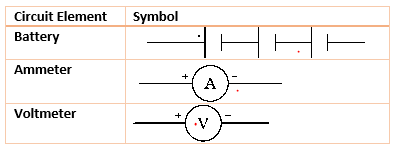Battery -> It is used to supply electric current to the circuit Ammeter -> It is used to measure electric current Voltmeter -> It is used to measure Potential difference in the circuit Question 24. What is meant by electrical resistance of a conductor? State how resistance of a conductor is affected when (i) a low current passes through it for a short duration (ii) a heavy current passes through it for about 30 seconds. Answer Electric resistance of a conductor is the obstruction offered by the conductor to the flow of the current through it. Electric resistance depends on lenght,area,resistivity and temperature.It does not depend on current. Infact current is determined by the voltage and resistance. So Resistance would be same in both the cases Question 25. How do we connect ammeter and voltmeter in an electric circuit? Draw a circuit diagram to justify your answer. What is likely to happen if the positions of these instruments are interchanged? Give reason. Answer Ammeter is connected in series while voltmeter is connected in Parallel. If the are interchanged,incorrect reading will be specifoed Question 26. Three resistors of 1 Ω, 2 Ω and 3 Ω are connected in parallel in a circuit. If 1 Ω resistor draw a current of 1 A, find the current through the other two resistors. Answer Potential difference accross 1 Ω resistor = IR=1 V Same potential difference will be there in other resistors also So Current in 2 Ω resistor= 1/2 =.5 A Similarly current in 3 Ω resistir= 1/3=.333 A Question 27. The resistance of a wire of 0.01 cm radius is 10 Ω. If the resistivity of the material of the wire is 50×10-8 ohm meters, find the length of the wire. Answer$R=\rho \frac {L}{A}$or$L = \frac {RA}{\rho}= \frac { 10 \times 3.14 \times 10^{-8}}{50 \times 10^{-8}}=.628 $m Question 28. Show different ways in which three resistance of R ohm each may be connected in a circuit. In which case is the equivalent resistance of the combination: (i) maximum (ii) minimum Answer When in Parallel , equivalent reistance$ \frac {1}{R_eq}=\frac {1}{R} + \frac {1}{R} + \frac {1}{R}$Req = R/3 When in Series, equivalent resistance Req = 3R, So when connected in series, maximum resistance is obtained and when connected in parallel ,minimum resistance is obtained Question 29. Establish a relationship to determine the equivalent resistance R of a combination of three resistors having resistance R1, R2 and R3 connected in series. Calculate the equivalent resistance of the combination of three resistors of 2 Ω, 3 Ω and 6 Ω joined in parallel. Answer$\frac {1}{R_eq}= \frac {1}{2} + \frac {1}{3} + \frac {1}{6} $Req= 1Ω Question 30. (i)State Joule's law of heating. (ii)Derive an expression for electric energy consumed in a device in terms of V, I and t, where V is the potential difference applied to it, I is the current drawn by it and t is the time for which the current flows? Question 31. a. What is meant by electric current? Name and define its SI unit. b. In a conductor electrons are flowing from B to A. What is the direction of conventional current? Give justification for your answer. c. A steady current of 1 ampere flows through a conductor. Calculate the number of electrons that flow through any section of the conductor in 1 second. (Charge on electron =$1.6 \times 10^{-19}$coulomb) Answer a. This flow of charge in metallic wire due to the potential difference between two conductors used is called electric current. It SI unit is Ampere 1 Ampere = 1 columb /1 sec b. Conventional current will flow from A to B as it is taken as the flow of positive charge c. We know 1 ampere meams 1 coulomb per sec. So we need to calculate the number of electrons in 1 coulomb$n= \frac {1}{1.6 \times 10^{-19}} = 6.25 \times 10^{18} \$